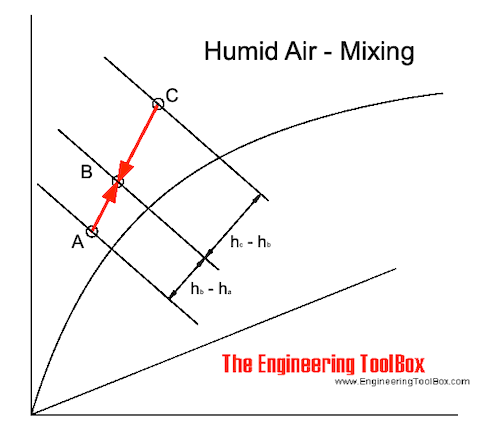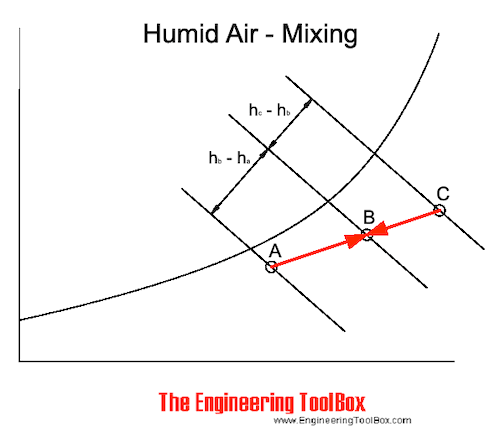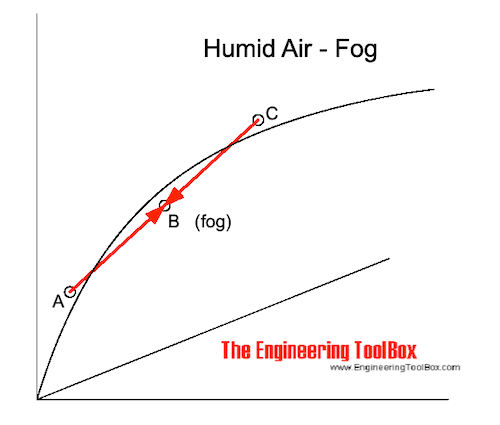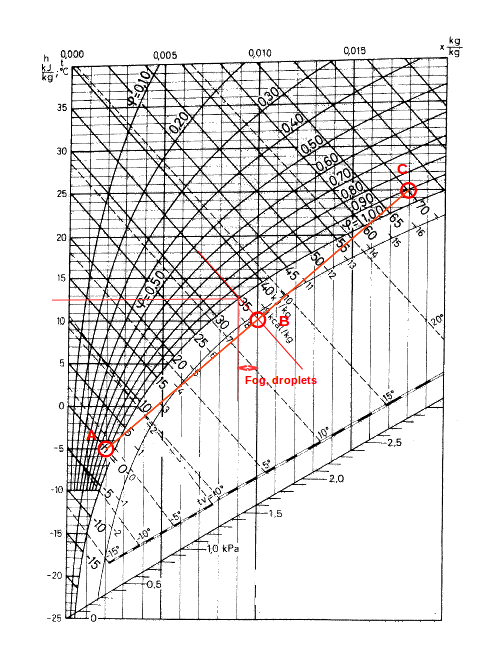Engineering ToolBox - Resources, Tools and Basic Information for Engineering and Design of Technical Applications!

# Mixing of Humid Air

## The change in state wwhen mixing moist air - enthalpy, heat, temperature and specific humidity.

In the Mollier diagram - or in the psychrometric chart - when mixing air of state A and C, the mixing point will be on a straight line in point B.

The position of point B depends on the air volume (or mass) of the air in state A and C.The mixing process are indicated in a Mollier diagram above and in a psychrometric chart below.### Enthalpy Balance when Mixing Air

The heat or enthalphy balance when humid air is mixed can be expressed as:

QA hA + QC hC = (QA + QC) hB                                   (1)

where

Q = volume of the air (m3)

h = enthalpy of the humid air (kJ/m3)

By transforming (1) the enthalpy of the mixed air can be expressed as:

hB = (QA hA + QC hC) / (QA + QC)                                      (1b)

Note! It's common to use air volume when calculating ventilation or air conditioning systems. Using the mass of air is more accurate but within the operation limits of common ventilation systems the volume approach is accurate enough. Be aware that this may not be true in industrial high temperature air handling processes - like dryers or similar.

### Moisture Balance when Mixing Air

The moisture balance when humid air is mixed can be expressed as:

QA xA + QC xC = (QA + QC) xB                                          (2)

where

x = specific humidity or humidity ratio (kgwater/kgdry_air)

By transforming (2) - the specific humidity of the mixed air can be expressed as:

xB = (QA xA + QC xC) / (QA + QC)                                       (2b)

### Humid Air and Fog

When hot humid air is mixed with cold air the result may be fog.

If the mixing point is below the saturation line - as indicated in the figure below - the moisture in the air condensates to small droplets floating in the air.For temperatures below 0oC the water droplets freezes to ice and snow is created.

### Resulting Temperature when Humid Air is Mixed

#### Mixing Point is above the Saturation Line

As long as the mixed air is above the saturation line (no fog in the mix) the temperature balance can be expressed by modifying the enthalpy balance like

QA cpa tA + QC cpa tC = (QA + QC) cpa tB                                           (3)

where

cpa = 1.01 - specific heat capacity of air at constant pressure (kJ/kgoC, kWs/kgK)

The temperature in the mix can be expressed by transforming (3):

tB = (QA tA + QC tC) / (QA + QC)                                               (3b)

#### Mixing Point is below Saturation Line

If the mixing point is below the saturation line - water is condensed as droplets and fog is created.

The amount of condense water can be estimated by following the constant enthalpy line from B to the saturation line. The condensate water is the difference between the specific humidity in point B and in the point where the enthalpy line crosses the saturation line.

The mixed temperature is where the enthalpy line crosses the saturation line.

### Example - Mixing Humid Air

#### Mixing Point is above the Saturation Line

1 m3 of air at 25oC and 50% relative humidity (C) is mixed with 1 m3 air at -5oC and 80% relative humidity (A).

From the Mollier diagram the humidity ratio of (C) is 0.0097 kg/kg. The enthalpy is 50 kJ/kg. The humidity ratio of (A) is 0.002 kg/kg. The enthalpy is 0 kJ/kg.

The humidity ratio of the mixture can be calculated like

xB = (QA xA + QC xC) / (QA + QC)                                             (4)

= ((1 kg) (0.002 kg/kg) + (1 kg) (0.0097 kg/kg) ) / ((1 kg) + (1 kg))

= 0.0058 kg/kg

The enthalpy of the mixed air can be calculated like

hB = ((1 kg) (0 kJ/kg) + (1 kg) (50 kJ/kg)) / ((1 kg) + (1 kg))

= 25 kJ/kg

The temperature of the mixed air can be calculated as:

tB = ((1 kg) (-5oC) + (1 kg) (25oC)) / ((1 kg) + (1 kg))

= 10oC

Based on the information above and the Mollier diagram - the relative humidity in the mixing point can be estimated to approximately 80%.

#### The Mixing Point is below the Saturation Line

1 m3 of air at 25oC and 90% relative humidity (C) is mixed with 1 m3 air at -5oC and 80% relative humidity (A).

From the Mollier diagram the humidity ratio of (C) is 0.018 kg/kg. The enthalpy is 70 kJ/kg. The humidity ratio of (A) is 0.002 kg/kg. The enthalpy is 0 kJ/kg.

The enthalpy of the mixture can be calculated as:

hB = ((1 kg) (0 kJ/kg) + (1 kg) (70 kJ/kg)) / ((1 kg) + (1 kg))

= 35 kJ/kg

The humidity ratio of the mixture can be calculated as:

xB = ((1 kg) (0.002 kg/kg) + (1 kg) (0.018 kg/kg)) / ((1 kg) + (1 kg))

= 0.01 kg/kg

Note! This is the humidity ratio including the water droplets - the fog.

The "virtual" mixing point in the Mollier diagram is where the enthalpy line - 35 kJ/kg and the humidity ratio line - 0.01 kg/kg - intersect.

The temperature in the mixed air can be found in the diagram where the enthalpy line - 35 kJ/kg - crosses the saturation line. From the Mollier diagram the mixed temperature is approximately 12.7 oC.

According the Mollier diagram the moisture ratio at this point is approximately  0.0089 kg/kg. The moisture created as fog or droplets is

(0.01 kg/kg) - (0.0089 kg/kg)

= 0.0011 kg/kg## Related Topics

• ### Air Psychrometrics

Moist and humid air - psychrometric charts, Mollier diagrams, air-condition temperatures and absolute and relative humidity and moisture content.

## Related Documents

• ### Air - Heating, Cooling, Mixing, Humidifying or Dehumidifying Processes

Basic air changing state processes - heating, cooling, mixing, humidifying and dehumidifying by adding steam or water - psychometric diagrams and the Mollier charts.
• ### Air - Humidifying by Adding Steam or Water

Air can be humidified by adding water or steam.
• ### Air - Humidifying with Steam, SI units

Using steam to humidify air.
• ### Air - Humidity Measurement from Dry and Wet Bulb Temperature

Relative humidity in moist air can estimated by measuring the dry and wet bulb temperature.
• ### Air - Humidity Ratio

The mass of water vapor present in moist air - to the mass of dry air.
• ### Air - Moisture Holding Capacity vs. Temperature

The moisture holding capacity of air increases with temperature.
• ### Air - Thermophysical Properties

Thermal properties of air at different temperatures - density, viscosity, critical temperature and pressure, triple point, enthalpi and entropi, thermal conductivity and diffusivity and more.
• ### Air and Steam Mixture

Air in the steam will lower the surface temperatures in heat exchangers - and less heat will be transferred.
• ### Air Heating Systems

Air heating buildings - heat supply vs. air flow and temperature.
• ### Cooling and Heating Equations

Latent and sensible cooling and heating equations - imperial units.
• ### Dry Bulb, Wet Bulb and Dew Point Temperatures

Dry Bulb, Wet Bulb and Dew Point temperatures can be used to determine the state of humid air.
• ### Heat Recovery Efficiency

Classification of heat recovery efficiencies - temperature efficiency, moisture efficiency and enthalpy efficiency - online heat exchanger efficiency calculator.
• ### Moist Air - Density vs. Water Content and Temperature

Density of the mix of dry air and water vapor - moist humid air.
• ### Moist Air - Enthalpy

Sensible and latent heat of moist air.
• ### Moist Air - Psychrometric Table for Pressure 29.92 inHg

Dry and wet bulb temperatures, saturation pressure, water vapor weight, specific volume, heat and more.
• ### Moist Air - Specific vs. Relative Humidity

Specific humidity of moist air vs. relative humidity, water vapor and air density.
• ### Moist Air - the Mollier Diagram

The Mollier diagram is a graphic representation of the relationship between air temperature, moisture content and enthalpy - and is a basic design tool for building engineers and designers.
• ### Moist Air - Transforming the Mollier Diagram to a Psychrometric Chart - or vice versa

The relationship between the psycrometric chart and the Mollier diagram.

## Engineering ToolBox - SketchUp Extension - Online 3D modeling!

Add standard and customized parametric components - like flange beams, lumbers, piping, stairs and more - to your Sketchup model with the Engineering ToolBox - SketchUp Extension - enabled for use with older versions of the amazing SketchUp Make and the newer "up to date" SketchUp Pro . Add the Engineering ToolBox extension to your SketchUp Make/Pro from the Extension Warehouse !

We don't collect information from our users. More about

## Citation

• The Engineering ToolBox (2004). Mixing of Humid Air. [online] Available at: https://www.engineeringtoolbox.com/mixing-humid-air-d_694.html [Accessed Day Month Year].

Modify the access date according your visit.

9.19.12